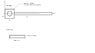# Solving Second Moment of Area for Beam w/ Missing Circle

• ganondorf29
In summary, the problem at hand is calculating the I value for a beam that changes from a 1" x 1" square to a 5" x 0.5" beam. The beam also has a missing circle in the front square, which needs to be accounted for. The formula for Ixx provided is incorrect, and the correct approach is to calculate I for the square without the hole and subtract the I for a cylinder. However, the resulting I will be about its own centroid, not the centroid of the beam, so the parallel axis theorem needs to be applied for the square and rectangular sections before adding them up. It is also important to determine whether the I value is needed about the x-axis or the y-axis

## Homework Statement

I'm having a problem calculating the I value for this beam. I'm not sure on how to account for the missing circle in the front square and how to account for the beam changing from a 1" x 1" square to a 5" x 0.5" beam

## Homework Equations

Ixx = bh^12/12

The centroid of the beam is around x = 2.89711 in

The thickness of the beam is the same and so is it's density

## The Attempt at a Solution

Here is a picture of the beam

**If there was no missing circle, could I just add I_square + I_rect = I_total? **

#### Attachments

•beam.jpg
9.1 KB · Views: 446
ganondorf29:
The reason why you need to calculate I (2nd moment of area) is not clear. Please include a complete statement of the problem you are trying to solve.

ganondorf29 said:

## The Attempt at a Solution

Here is a picture of the beam

**If there was no missing circle, could I just add I_square + I_rect = I_total? **

First thing, just get I for the square (with no hole in it) and then subtract the I for a cylinder. That will give you the I for the shape.

Second thing: The I that you get will be about its own centroid, not the centroid of the beam. So you need apply parallel axis theorem for the square (with the hole) and the rectangular section before you add them up.

Do you want the I about the x-axis, or the y-axis? If about the x-axis, the parallel axis theorem as suggested by rockfreak does not apply. You made a mistake in your formula for Ixx in post #1. Can you see what the error is?

rock.freak667 said:
First thing, just get I for the square (with no hole in it) and then subtract the I for a cylinder. That will give you the I for the shape.

Second thing: The I that you get will be about its own centroid, not the centroid of the beam. So you need apply parallel axis theorem for the square (with the hole) and the rectangular section before you add them up.

EDIT: Pongo is right, forget my parallel axis thing, I keep substituting I with J.